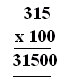Email us to get an instant 20% discount on highly effective K-12 Math & English kwizNET Programs!

#### Online Quiz (WorksheetABCD)

Questions Per Quiz = 2 4 6 8 10

### Grade 3 - Mathematics9.10 Multiplication Review Test

 Q 1: 7482 x 4Answer: Q 2: In the multiplication problem shown what are the fators?315 and 31500100 and 31500315 and 10031500 Q 3: 16 x 3 = Answer: Q 4: 342 x 4Answer: Q 5: In the multiplication problem shown what is the product?12036156 Q 6: Expand 23,5682,000+300+50+60+820,000+300+500+60+820,000+3,000+500+60+8 Q 7: 7432 x 6Answer: Q 8: In the multiplication problem shown what is the product?22366 Question 9: This question is available to subscribers only! Question 10: This question is available to subscribers only!

#### Subscription to kwizNET Learning System offers the following benefits:

• Unrestricted access to grade appropriate lessons, quizzes, & printable worksheets
• Instant scoring of online quizzes
• Progress tracking and award certificates to keep your student motivated
• Unlimited practice with auto-generated 'WIZ MATH' quizzes